# Texas Go Math Grade 3 Lesson 12.3 Answer Key Divide by 5

Refer to our Texas Go Math Grade 3 Answer Key Pdf to score good marks in the exams. Test yourself by practising the problems from Texas Go Math Grade 3 Lesson 12.3 Answer Key Divide by 5.

## Texas Go Math Grade 3 Lesson 12.3 Answer Key Divide by 5

Essential Question
What does dividing by 5 mean?

Unlock the Problem

Kaley wants to buy a new cage for Coconut, her guinea pig. She has saved 35¢. If she saved a nickel each day, for how many days has she been saving?

• How much is a nickel worth?

One Way
Count up by 5s.

• Begin at O.
• Count up by 5s until you reach 35.
• Count the number of times you count up.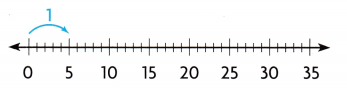You counted up by 5 seven times. 35 ÷ 5 = _7__
So, Kaley has been saving for _7__ days.

Another Way
Count back on a number line.

• Start at 35.
• Count back by 5s until you reach 0.
Complete the jumps on the number line.
• Count the number of times you jumped back 5.You jumped back by 5 _7__ times.
35 ÷ 5 = _7__

Math Talk
Mathematical Processes
What if Kaley saved 7¢ each day instead of a nickel? What would you do differently to find how many days she has
saved?

Strategy for Dividing with 5
You have learned how to use doubles to multiply. Now you will learn how to use doubles to divide by 5.

Divide by 10, and then double to divide by 5.
When the divisor is 5 and the dividend is even, you can use a 10s fact.
First, divide by 10.
After you divide, double the quotient.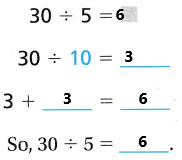Share and Show

Math talk
Mathematical Processes
Explain how counting up to solve a division problem is like counting back on a number line.

Question 1.
Count back on the number line to find 15 ÷ 5. ____Use count up or count back on a number line to solve.
Explanation: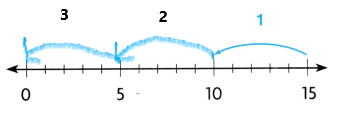Count back on a number line.

• Start at 15.
• Count back by 5s until you reach 0.
• Complete the jumps on the number line.
• Count the number of times you jumped back 3.

Question 2.
10 ÷ 2 = ___Explanation: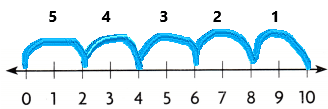Count back on a number line.

• Start at 10.
• Count back by 2s until you reach 0.
• Complete the jumps on the number line.
• Count the number of times you jumped back 5.

Question 3.
20 ÷ 5 = ___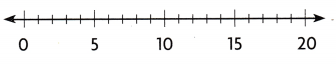Explanation:Count back on a number line.

• Start at 20.
• Count back by 2s until you reach 0.
• Complete the jumps on the number line.
• Count the number of times you jumped back 4.

Find the quotient.

Question 4.
50 ÷ 5 = ___
Explanation: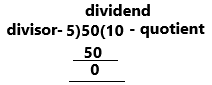There are three things in division. They are dividend, divisor, and quotient.
50 is the dividend.
5 is the divisor.
If we solve both 50 by 5 we get 10.
Therefore 10 is the quotient.

Question 5.
5 ÷ 5 = ___
Explanation:
There are three things in division. They are dividend, divisor, and quotient.
5 is the dividend.
5 is the divisor.
If we solve both 5 by 5 we get 1.
Therefore 1 is the quotient.

Question 6.
45 ÷ 5 = ___
Explanation:
There are three things in division. They are dividend, divisor, and quotient.
45 is the dividend.
5 is the divisor.
If we solve both 45 by 5 we get 9.
Therefore 9 is the quotient.

Algebra Complete the table.

Question 7.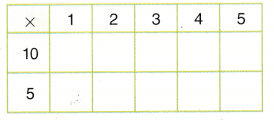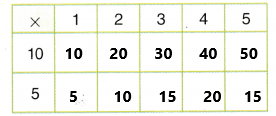Explanation:
This table indicates multiplication.
10*1=10, 10*2=20, 10*3=30, 10*4=40, 10*5=50.  {10th table up to 5 times}.
5*1=5, 5*2=10, 5*3=15, 5*4=20, 5*5=25. {5th table up to 5 times}.

Question 8.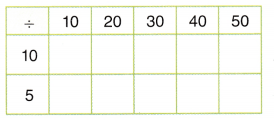Explanation:
This table indicates the division (÷).
10÷10=1, 20÷10=2, 30÷10=3, 40÷10=4, 50÷10=5 { divide by 10}.
10÷5=2, 20÷5=4, 30÷5=6, 40÷5=8, 50÷5=10 { divide by 5}.

Problem Solving

Question 9.
Evaluate Guinea pigs eat hay, pellets, and vegetables. If Wonder Hay comes in a 5-pound bag and costs $15, how much does 1 pound of hay cost? Answer: The answer is 3 Explanation: Given: Guinea pigs were used to eat hay, pellets, vegetables. If the hay comes in a 5-pound bag and it costs 15$. Then we need to find out the 1 pound of hay cost.
so, let’s take 1 pound of hay cost is ‘X’ .
we already know that a 5-pound bag cost is 15$. We can write this as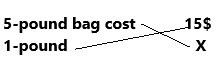Cross multiply, this we can write it as 5X=15$
X=15/5
X=3.
Therefore, 1 pound of hay cost is 3$. Question 10. H.O.T. The pet store has 20 guinea pigs and plans to put a pair of guinea pigs in each cage. If the pet store has 8 cages, how many more cages will the pet store need? Answer: 2 cages. Explanation: The total number of guinea pigs that pet stores have=20. They are planning to put the guinea pigs in a paired way, which means each pair has 2 pigs. Already pet store has 8 cages to put paired pigs. Now we need to find out the number of cages will pet stores need. By diagrammatically,By mathematically, 20/2=10 pairs, we already have 8 cages so, 10-8=2 cages. Pet stores need 2 cages. H.O.T. Pose a Problem Question 11. Maddie went to a veterinary clinic. She saw the vet preparing some carrots for the guinea pigs. Write a division problem that can be solved using the picture of carrots.Answer: 2 carrots Explanation:Question 12. Multi-Step Write a similar problem by exchanging the number of equal groups and numbers in each group. Solve the problem. Describe what the quotient in your problem represents. Answer: Explanation:We already know there are 2 groups and 5 in each group so, we get 5*2=10 carrots are present totally. From the above diagram, the total is 10. But the question asked was about the quotient. so, total/amount in each group which means 10/5=2Daily Assessment Task Fill in the bubble for the correct answer choice. You may use a division strategy to help you solve. Question 13. Analyze Beth keeps her rock collection in boxes. She has 30 rocks. She puts 5 rocks in each box. How many boxes of rocks does Beth have? (A) 5 (B) 6 (C) 7 (D) 8 Answer: Option B is correct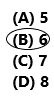Explanation: Total number of rocks Beth keeps with her=30 Number of rocks she puts in each box=5 Now we need to find out the number of boxes is Beth is having=30÷5=6. Therefore, 6 boxes are present. Question 14. Hasib pays$50 for new T-shirts. Each T-shirt costs $5. How many T-shirts does Hasib buy? (A) 5 (B) 4 (C) 2 (D) 10 Answer: Option D is correct.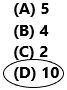Explanation: Total amount Hasib pays for new T-shirts=50$

cost of each T-shirt=5$We need to find out the number of T-shirts did he buy=50/5=10. Therefore, 10 T-shirts Hasib buys. Question 15. Multi-Step Ana picks 25 apples. Pedro picks 20 apples. Ana and Pedro use the apples to make apple pies. They put 5 apples in each pie. How many apple pies do they make? (A) 4 (B) 5 (C) 9 (D) 8 Answer: Option C is correct.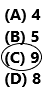Explanation: The number of apples Ana pick=25. The number of apples Pedro pick=20. Both are used their apples to make apple pies and they put 5 apples in each pie. Here we need to find out the number of apple pies they are done. By simplifying this, total apples=25+20=45. In each pie they put 5 apples, from this we can get the number of apple pies=45/5=9. Therefore, 9 apple pies they make. Texas Test Prep Question 16. Guinea pigs sleep about 45 hours every 5 days with their eyes open. About how many hours a day do guinea pigs sleep? (A) about 5 hours (B) about 40 hours (C) about 9 hours (D) about 50 hours Answer: Option C is correct.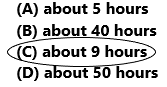Explanation: The total number of hours guinea pigs sleep with their eyes open=45. The number of days they sleep=5. We need to find out the number of hours a day does guinea pigs sleep=45/5=9. Therefore, they sleep 9 hours a day. ### Texas Go Math Grade 3 Lesson 12.3 Homework and Practice Answer Key Use count up or count back on a number line to solve. Question 1. 30 ÷ 5 = ___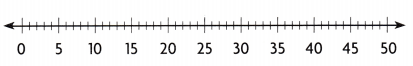Answer: 6Explanation: Count up by 5s. • Begin at O. • Count up by 5s until you reach 30. • Count the number of times you count up. You counted up by 5 six times. 30 ÷ 5 = _6_. Question 2. 45 ÷ 5 = ___Answer: 9 Explanation: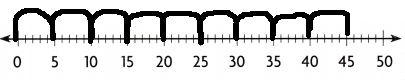Count up by 5s. • Begin at O. • Count up by 5s until you reach 45. • Count the number of times you count up. You counted up by 5 nine times. 45 ÷ 5 = _9_. Problem Solving Question 3. A librarian has 35 books on a cart. He evenly separates the books on five shelves. How many books are on each shelf? Answer: 7 Explanation: The number of books a librarian has on a cart=35. The number of shelves he has separated for books=7. The number of books on each shelf=35/5=7. Therefore, 7 books on each shelf. Question 4. There are 25 paint cans. If the art teacher puts five cans in each box, how many boxes does she need? Answer: 5 Explanation: The number of paint cans=25. The number of cans the art teacher puts in each box=5. The number of boxes she needs to be kept=25/5=5. Therefore, 5 boxes are needed. Question 5. Marky picks some flowers. Write a division problem that can be solved using the picture of the flowers. Then solve the problem.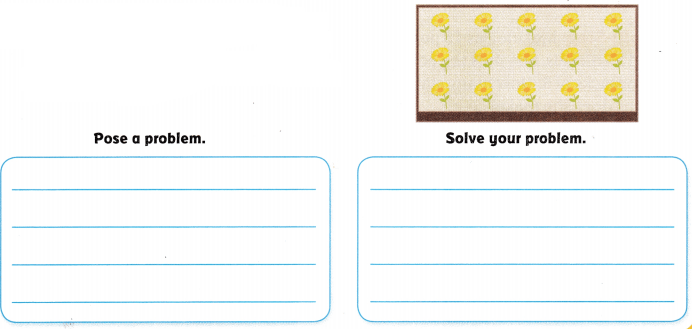Answer: 5 flowers Explanation: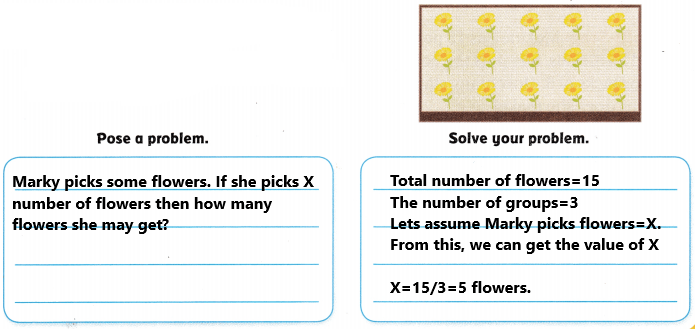Texas Test Prep Lesson Check Fill in the bubble completely to show your answer. Question 6. Dikembe has 25 toy boats. He puts ‘them in groups of 5. How many boats are in each group? (A) 5 (B) 6 (C) 20 (D) 10 Answer: Option A is correct.Explanation: The number of toy boats Dikembe has=25. The number of groups he has put=5 We need to find out the number of boats in each group=25/5=5. Therefore, 5 boats in each group. Question 7. Jeri pays$30 for CDs. Each CD costs $5. How many CDs does Jeri buy? (A) 5 (B) 6 (C) 25 (D) 15 Answer: Option B is correct.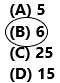Explanation: The number of CDs did Jeri pay=30$
Cost of each CD=5\$
The number of CDs did Jeri buy=30/5=6.
Therefore, he bought 6 CDs.

Question 8.
Damian has 20 crayons. There are 5 crayons in each box. How many boxes of crayons does Damian have?
(A) 5
(B) 4
(C) 15
(D) 10Explanation:
The number of crayons Damian has=20
The number of crayons in each box=5
The number of boxes does Damian have=20/5=4.
Therefore, he has 4 boxes.

Question 9.
A group of 45 people go camping. Each tent sleeps 5 people. How many tents will the campers need?
(A) 7
(B) 8
(C) 9
(D) 50Explanation:
The number of people going camping=45
The number of people sleeping in each tent=5
The number of tents the campers needed=45/5=9.
Therefore, They need 9 tents.

Question 10.
Multi-Step Maxine has 15 pennies and 25 nickels. She puts the coins into stacks of 5. How many stacks of coins does she make?
(A) 5
(B) 8
(C) 3
(D) 9Explanation:
The number of pennies and nickles Maxine has=12+25=40
The number of coins she puts in stacks=5
The number of stacks she made=40/5=8.

Question 11.
Multi-Step There are 17 boys and 13 girls in gym class. They are in 5 equal teams. how many students are on each team?
(A) 10
(B) 5
(C) 30
(D) 6